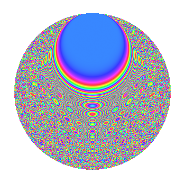# Properties

 Label 3234.2.bjLevel 3234 Weight 2 Character orbit bj Rep. character $$\chi_{3234}(19,\cdot)$$ Character field $$\Q(\zeta_{30})$$ Dimension 640 Sturm bound 1344

# Related objects

## Defining parameters

 Level: $$N$$ $$=$$ $$3234 = 2 \cdot 3 \cdot 7^{2} \cdot 11$$ Weight: $$k$$ $$=$$ $$2$$ Character orbit: $$[\chi]$$ $$=$$ 3234.bj (of order $$30$$ and degree $$8$$) Character conductor: $$\operatorname{cond}(\chi)$$ $$=$$ $$77$$ Character field: $$\Q(\zeta_{30})$$ Sturm bound: $$1344$$

## Dimensions

The following table gives the dimensions of various subspaces of $$M_{2}(3234, [\chi])$$.

Total New Old
Modular forms 5632 640 4992
Cusp forms 5120 640 4480
Eisenstein series 512 0 512

## Trace form

 $$640q - 80q^{4} + 24q^{5} - 80q^{9} + O(q^{10})$$ $$640q - 80q^{4} + 24q^{5} - 80q^{9} - 8q^{11} + 12q^{15} + 80q^{16} - 60q^{17} + 12q^{22} - 8q^{23} - 108q^{25} + 24q^{26} + 40q^{29} - 18q^{31} + 6q^{33} - 160q^{36} + 36q^{37} - 24q^{38} - 30q^{40} - 12q^{44} + 24q^{45} - 48q^{47} - 40q^{51} - 10q^{58} - 4q^{60} + 60q^{61} + 160q^{64} - 64q^{67} + 60q^{68} + 144q^{71} + 180q^{73} + 40q^{74} - 24q^{75} + 60q^{79} + 36q^{80} + 80q^{81} + 96q^{82} + 280q^{85} + 36q^{86} + 26q^{88} + 48q^{89} - 16q^{92} + 40q^{93} + 20q^{95} - 16q^{99} + O(q^{100})$$

## Decomposition of $$S_{2}^{\mathrm{new}}(3234, [\chi])$$ into newform subspaces

The newforms in this space have not yet been added to the LMFDB.

## Decomposition of $$S_{2}^{\mathrm{old}}(3234, [\chi])$$ into lower level spaces

$$S_{2}^{\mathrm{old}}(3234, [\chi]) \cong$$ $$S_{2}^{\mathrm{new}}(77, [\chi])$$$$^{\oplus 8}$$$$\oplus$$$$S_{2}^{\mathrm{new}}(154, [\chi])$$$$^{\oplus 4}$$$$\oplus$$$$S_{2}^{\mathrm{new}}(231, [\chi])$$$$^{\oplus 4}$$$$\oplus$$$$S_{2}^{\mathrm{new}}(462, [\chi])$$$$^{\oplus 2}$$$$\oplus$$$$S_{2}^{\mathrm{new}}(539, [\chi])$$$$^{\oplus 4}$$$$\oplus$$$$S_{2}^{\mathrm{new}}(1078, [\chi])$$$$^{\oplus 2}$$$$\oplus$$$$S_{2}^{\mathrm{new}}(1617, [\chi])$$$$^{\oplus 2}$$

## Hecke characteristic polynomials

There are no characteristic polynomials of Hecke operators in the database